All submissions of the EM system will be redirected to Online Manuscript Submission System. Authors are requested to submit articles directly to Online Manuscript Submission System of respective journal.

# Research

, Volume: 11( 5) DOI: 10.37532/2320-6756.2023.11(5).349

## Four-dimensional model of space

*Correspondence:
V.A. Tokarev All-Russia Scientific Research Institute “Gradient”, Rostov-on-Don 344000, Russia , E-mail: tokarevvalerij@yandex.ru

Received date: 13-April-2023, Manuscript No. tspa-23-95511; Editor assigned: 18-April-2023, Pre-QC No. tspa-23-95511 (PQ); Reviewed: 1May-2023, QC No. tspa-23-95511 (Q); Revised: 10-May-2023, Manuscript No. tspa-23-95511 (R); Published: 27-May-2023, DOI. 10.37532/2320-6756.2023.11(5).349

Citation: Tokarev V. A. Four-Dimensional Model of Space. J. Phys. Astron.2023;11(5):349.

### Abstract

Time is our perception of the movement of a closed three dimensional Universe in the direction of the orthogonal fourth spatial dimension due to its own expansion. Such a hypothesis makes it possible to explain the reason for the limit of the speed of light, the mechanism of cosmological time dilation, time dilation when moving at high speeds and in strong gravitational fields, as well as the explanation of cosmological red shift without involving the Doppler Effect. Matter can be represented in the form of electromagnetic waves rolled into a ring, which explains the particle-wave dualism of matter, the quantum properties of the microcosm and the paradoxes of the spin of elementary particles. It is possible to explain the apparent accelerated expansion of the Universe without the participation of dark energy, as well as the excess of the estimated mass of galaxies over visible one without the participation of dark matter. The presented model allows us to estimate the radius of a closed Universe of 1.1 Gpc, the rate of expansion of the Universe - 80,000 kms-1, and the speed of propagation of relict background radiation - 80 kms-1. Incredibly, such a radical hypothesis allows for a fairly simple and repeatable experimental test based on measuring the propagation speed of background radiation, which was successfully done later.

### Keywords

Large-scale structure of the universe; Cosmological parameters; Dark energy; Dark matter

### Introduction

The multidimensionality of our space is now generally recognized. Four-dimensional spaces are known in which the fourth dimension -time-is a special physical entity orthogonal to the three spatial dimensions . One of the theories explaining the structure of our world is the theory of superstrings . The main provisions of this theory are the multidimensionality of our space and the representation of elementary particles as modes of vibrations of superstrings. Superstring theory assumes that there are three extended spatial dimensions available to our perception, seven microscopic dimensions and time. A similar idea of the multidimensionality of our space is proposed in this article, however, unlike existing theories, it tries to completely eliminate the differences between space and time.

Space and time

Suppose that our Universe is a three-dimensional sphere-like closed space P3(x,y,z) expanding from a point O in a fourdimensional space P4(x,y,z,t) (FIG. 1) . All objects iN of our Universe are rigidly bound to three-dimensional space and, regardless of the state of rest or movements relative to this space, move in the direction of the fourth dimension due to the pansion of the Universe. We perceive this movement as the passage of time. The rate of expansion of spaceSin this case corresponds to the distance by which the radius of the Universe R has increased during the time that we perceive as 1 second. To a stationary observer N A moving with the space along the axis Ot0 from point O1 to pointA (FIG. 1), it should seem that time for an observer NB moving at the speed of V from point O1 to the point B, flows slower, since the projection t3 of the point B on the time axis associated with the observer N Ais in the past. The time t3 - t1 can be determined by the equation

Figure 1: A three-dimensional space P3(x,y,z) expanding from a point Oin a four-dimensional space P4(x,y,z,t): Ot0-the time axis;R-the radius of the Universe; S-the rate of expansion of the Universe; iM-objects having mass; V, c-object speed; d-distance between objects; βi -the angle of entry of radiation into space; ψi-the angle of inclination of the spacRelative velocity of time flow for moving NB and stationary N A observers (t3 - t1)/(t2 - t1)does not depend on the radius of space R=nS, where n is the number of seconds that have passed since the beginning of the expansion of the Universe, is valid for any region of space with constant curvature and differs slightly from that calculated by the Lorentz formula .

he vector of the maximum possible speed Vmax= c is directed tangentially to three dimensional space at the starting point O1, and the projection on the time axis Otoof the point C to which the observer Nc moved, does not change: t3 - t1=0, i.e. from the point of view of the observer N A , time for the observer C N stops (FIG. 1). From the point of view of the observer Nc time at pointA has stopped, and the observer’s own time at point C flows normally. The resulting time dilation is determined by the final location of the observers and does not depend on which of them was moving. According to the general theory of relativity, the space time continuum has a hyperbolic character . This suggests that objects with masses Mibend space inversely proportional to the distance from them by 1/xi , forming around themselves a region with curvature Ki ,Where 0 k- the coefficient of elasticity of the space; xi - the distance from the center of the object, normalized relative to points of maximum curvature. As a result, while the flow of universal time0 t, determined by the expansion of space, is uniform, each ith point of space has its own local time txi , the speed of which is determined by the angle of inclination Φi of the curved space relative to the vector of its expansion at this point xi (FIG. 1). For a region of space with curvature Ki, the velocity of local time txitrelative to the world one 0 t is determined by the equationThe Speed of Light

The speed of light c under accepted assumptions is determined by the ratio of the magnitude of displacement in three-dimensional space 1 OC to the magnitude of displacement in the direction of the fourth dimension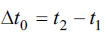(FIG. 1).The maximum value of the speed of light is determined by the curvature of free space and isNear massive objects, the speed of light is also limited by the slope of space ψi, which, for a fixed radius of curvature Ri, reduces the speed of light for various βi proportionally to cosψi . The resulting dependence of the speed of light cix on the radius of curvature of space Ri and the angles βi and ψi when moving along thexaxis can be represented as,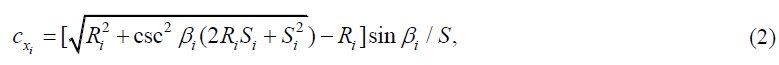where S Scosψi

The radius of curvature of space in the plane of ray propagation at the point Ni can be determined by the equation ,where xi, yi are the relative coordinate values of the point Ni. The angle of inclination of the space ψi can be determined by the equation,Graphs of the dependence of cxi relative to the rate of expansion of space S when moving along the x axis from the distance yi from the center of the object are shown in FIG. 2. The dependence of the speed of light on the curvature of space fully corresponds to the basic provisions of the general theory of relativity, in particular, confirms the presence of the Shapiro effect within the framework of the presented model of space, and leads to a curvature of the trajectory of the light beam [1,5]. The instantaneous values of the angle of rotation per unit length of the path of the light beam can be calculated by the equation,

Figure 2: The speed of light cxi relative to the rate of expansion of space Sfor different iy at βi=10°.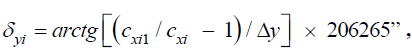where cxi1 and cxi are the speeds of light rays spaced Δy, calculated by equation (2).

To estimate the final form of the trajectory of the light beam, we can use the specific values of the rotation angle δi0 when the beam passes at the minimum distance from the object (ix = 0), which are shown in FIG. 3. At βi>0.003°, the beam trajectories are always open, and at βi<0.003°, it is possible to capture a beam of light, at which it remains in orbit around an object with mass. For example, with βi=0.001°, this happens when the beam passes at a relative distance of iy from about 1 to 4. The presence of two points of intersection of the curve of the specific angle of rotation 0iδ and the curve of the specific angle of rotation when moving around the circle δ0, equal to 1/iy [rad], automatically keeps the beam in the area of the point yi≈1, and leaves the opportunity to leave the orbit with random perturbations of the curvature of space when the beam is in the area of the point yi≈4. As βi decreases, the point of intersection of the curves on the graph moves to infinity, which means that in a relatively flat space, the probability of transition of all rays to closed orbits tends to 1. Thus, initially there were only extremely high-frequency electromagnetic oscillations in the Universe; the energy density and uniformity of space were very high. With the expansion of space, the possibility of its local deformation appeared due to its own energy of short-wave oscillations. Further, the deformation zones became centers of attraction and capture of other electromagnetic waves, which, falling into closed orbits, formed a semblance of standing waves. As a result, local areas of concentration of electromagnetic energy or what is now called matter were formed. For the first time such an idea was formulated by Albert Einstein in ref. .

Figure 3: Specific values of the rotation angle δ10Specific values of the rotation angle yi Specific values of the rotation angle βi and when moving around a circle δ0

An integer number of wavelengths in these orbits further determined the quantum properties of the microcosm. At a sufficiently small distance, the particles of matter interacted with each other, generating electromagnetic oscillations with new frequencies on the nonlinearity of the curved space. The resulting relatively low-frequency oscillations could not form closed orbits and remained in the form of radiation. High-frequency vibrations formed new stable particles of matter. Thus, the separation of matter and electromagnetic radiation occurred. It should also be noted that the axis of rotation of electromagnetic waves forming particles of matter lies outside our three-dimensional space, which can cause paradoxes of the spin of elementary particles. The propagation of light at the exit angle i from the point of formation continues until it enters a strongly curved space, for example, when it hits a particle of matter. As the light beam propagates, the angle of entry into space is constantly increasing, reaching the limit value of βiii=90°. The speed of light drops to the speed of expansion of space, and from the point of view of a three-dimensional observeri N, tends to zero, while the wavelength increases. Consequently, all received signals experience a red shiftwhich depends only on the angles αi and ψi (FIG. 4). Accordingly, from the point of view of each of the two fixed observers separated by αi, the time of the other flows slower by 1+z times. It follows from modern concepts that the Universe expanding after the Big Bang became transparent at a temperature of about 3000 K. Now the relict background radiation has a temperature of the order of 3 K, hence its red shift is1000z, which corresponds to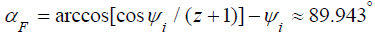and a 1000-fold increase in the size of the Universe in time for ψi≈0.

Electromagnetic waves forming matter are relatively stationary and do not experience red shift when space expands. Considering their energy E=mc2 unchanged, we can conclude that the mass of matter decreases with the expansion of space. In the limit, perhaps, the mass of the particle will not be enough to warp space and it will unfold into a propagating electromagnetic wave, which will eventually fade out in the same way as the relict radiation.

Dimensions of the Universe

To determine the size of the Universe, we can use the known value of the deviation of the radio emission of a quasar when it is covered by the Sun, which is 1.75” . FIG. 5 shows the total values of the rotation angle σyi with the curvature of the space of the form1/x, calculated by the equation

Figure 5: The total values of the rotation angle σyi when the beam passes at a distance of yi from the object for differenti βi.From the equation (3) and the graphs in FIG. 4 it follows that for all known quasars with z≥0.16, the angle of entry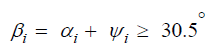which allows us to determine the total angle of rotation along the curve for βi= 90°(FIG. 5). Modeling of the curvature of space created by the Sun taking into account the density increase as it approaches its center allows us to conclude that the curvature of space on the surface of the Sun coincides with a curve of the form 1/x. Therefore, iy of the Sun’s surface can be taken to be equal to yss=1.92 (FIG. 5).

The angle of the luminous flux from the surface of the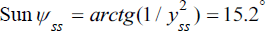from which it follows that when light propagates from the Sun to the Earth at a distance of dEF(FIG. 1), the Earth is moving in the direction of the fourth dimension at a distance ofi.e. the rate of expansion of space 78500Skms-1. The obtained value with a modern estimate of the Hubble constant 72 kms-1Mpc-1 corresponds to the radius of the universe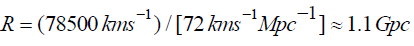. To compare the results obtained with the observational data, assume that all galaxies consist of stars on average similar to the Sun, i.e. tha ψis=15°. FIG. 6 graphs of the dependence of the red shift zon the distance to the object d are given, calculated using the equations (3), (4)for H=72KMs-1Mpc-1. Curve 1 according to the proposed model at ranges up to 300 Mpc coincides with curve 3 calculated by the equation (5). At distances of more than 300 Mpc the curves diverge sharply, and curve 3 is confirmed by observations of type Ia supernova (SN Ia). The indicated divergence of curves 1 and 3 may be due to the combined action of the following factors:

• As follows from Fig. 4, at a fixed αi, the red shift strongly depends on the radiation output angle ψi. The angle of radiation output from a rarefied gas cloud ψSN is significantly smaller than the angle of radiation output from incomparably dense stars (ψi=15°), and it can be considered equal to 0°. Accordingly, the red shift of the galaxy z and the supernova 0 z that erupted in it may differ by about 2 times (FIG. 6, curves 1, 2). As a result, the integral luminosity of a SN Ia in the visible part of the spectrum, calculated by the Planck formula, in the red shift range of galaxies z from 0.1 to 1.5 is less than expected by Iz=1.267-3.241 .

Figure 6: Dependence of the red shift z on the distance to the object d.

• For an observer on Earth, time in the galaxy where the SN Ia broke out flows according to equation (3) 1+z times slower. Accordingly, the observed luminosity of SN Ia decreases by 1+z0 times. At z = 0.1-1.5, the luminosity drops by It=1.301-1.745 times. An increase in the duration of a supernova outbreak with an increase in red shift is confirmed by observations.

The dependence of the radiation intensity on the speed of light according to the Planck formula leads to the fact that at temperatures above 30, 000 K, the radiation intensity in the visible part of the spectrum decreases slightly with a decrease in the speed of light. The speed of light in accordance with equation (1) falls for SN Ia in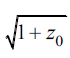times. The corresponding intensity reduction values for z=0.1-1.5 are Ic=1.021-1.145.

• The mass of matter increases with the fall of the speed of light, therefore, the achievement of the critical mass for a SN Ia (the Chandrasekhar limit) occurs with fewer atoms, respectively, fewer photons of radiation are formed during the explosion. The decrease in luminosity can be estimated at Im=1.031-1.743.

• The difference in the emission angles of the galaxy and the gas cloud SN Ia means that at the time of the explosion, the star was at a smaller distance than that determined for the previously observed galaxy. The distance reduction is determined by the value KR=cosψis=cos15°=0.966.

The resulting decrease in the visible luminosity of SN Ia compared to the expected for curve 1 is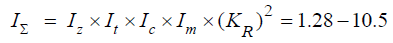times, which is equivalent to an increase in apparent range by 1.13 to 3.24 times. Curve 4 of apparent range up to SN Ia, recalculated from curve 1 taking into account the above corrections, is shown in FIG. 6. It coincides well with the curve 3, determined by the visible luminosity of SN Ia for H=72 kms-1Mpc-1, up to distances of the order of 1500 Mpc (0.5z). Then curves 3 and 4 diverge, and the visible luminosity corresponds to curve 4. Modern physics interprets this as an accelerated expansion of the Universe due to the action of dark energy, however, the effect can be explained without involving new entities. The proposed model allows us to solve another problem - the existence of dark matter. As follows from equation (2), the speed of light ix c largely depends on the curvature of space and grows in direct proportion toand inversely proportional to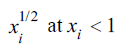. The result of modeling the curvature of space created by the stars of the galaxy, taking into account the possible density of their distribution, shown in FIG. 7 (simulation result for 10000 points), showed that the radius of curvature of space at the edge of the galaxy can be 10 times to 20 times smaller than in neighboring regions, which it leads to a drop in the speed of light and a proportional increase in the mass of the stars located there . The number of stars located on the periphery of the galaxy can reach 15% to 30% of the total number and equivalent total mass of the galaxy can be 2 times to 7 times greater than the visible one. At the same time, the peripheral mass will affect mainly the external interaction of galaxies, which is actually observed . The assumption made about the physical nature of time admits experimental verification - it is possible to directly measure the rate of expansion of spaceS. To do this, it is necessary to determine the delay timek T, which provides the maximum of correlation function of background radiation signals received from one direction in space by receivers spaced along the same direction at a distance of .r Indeed, since the angle of entry into the space for background radiation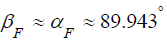is defined, then, which means that at present the rate of expansion of the Universe is about a thousand times greater than the rate of propagation of relict radiation.

Figure 7: The profile of the relative deflection of space depending on the distance L to the center of the galaxy.

### Conclusion

A four-dimensional model of space is considered in which a closed three dimensional Universe moves in the direction of the fourth dimension due to its own expansion, and this movement is perceived in three-dimensional space as time. Within the framework of the presented model, it is possible to explain the reason for the limit of the speed of light, the mechanism of cosmological time dilation, time dilation when moving at high speeds and in strong gravitational fields, as well as an explanation of the cosmological red shift without involving the Doppler Effect. One of the main consequences of the assumption made about the physical essence of time is the possibility of representing matter in the form of electromagnetic waves rolled into a ring, which explains the particle-wave dualism of matter, the quantum properties of the microcosm and the paradoxes of the spin of elementary particles. The presented model allows us to estimate the radius of a closed Universe of 1.1 Gpc, the rate of expansion of the Universe - 80,000 kms-1, and the rate of propagation of relict background radiation 80 kms-1. It is possible to explain the apparent accelerated expansion of the Universe without the involvement of dark energy, as well as the excess of the estimated mass of galaxies over the visible one without the involvement of dark matter. The presented model does not contradict the basic provisions of the general theory of relativity, and the assumption about the physical essence of time allows for a fairly simple experimental verification based on measuring the propagation speed of relict background radiation.

### REFERENCES

Welcome Message

Select your language of interest to view the total content in your interested language

###### Citations : 291

Journal of Physics & Astronomy received 291 citations as per Google Scholar report

#### Indexed In

• Open J Gate
• China National Knowledge Infrastructure (CNKI)
• Cosmos IF
• Directory of Research Journal Indexing (DRJI)
• MIAR
• Secret Search Engine Labs
• Euro Pub
• clarivate-web-of-science-logo-vector
• ICMJE

View More

Berlin, Germany

### World Congress on Quantum Physics

Berlin, Germany